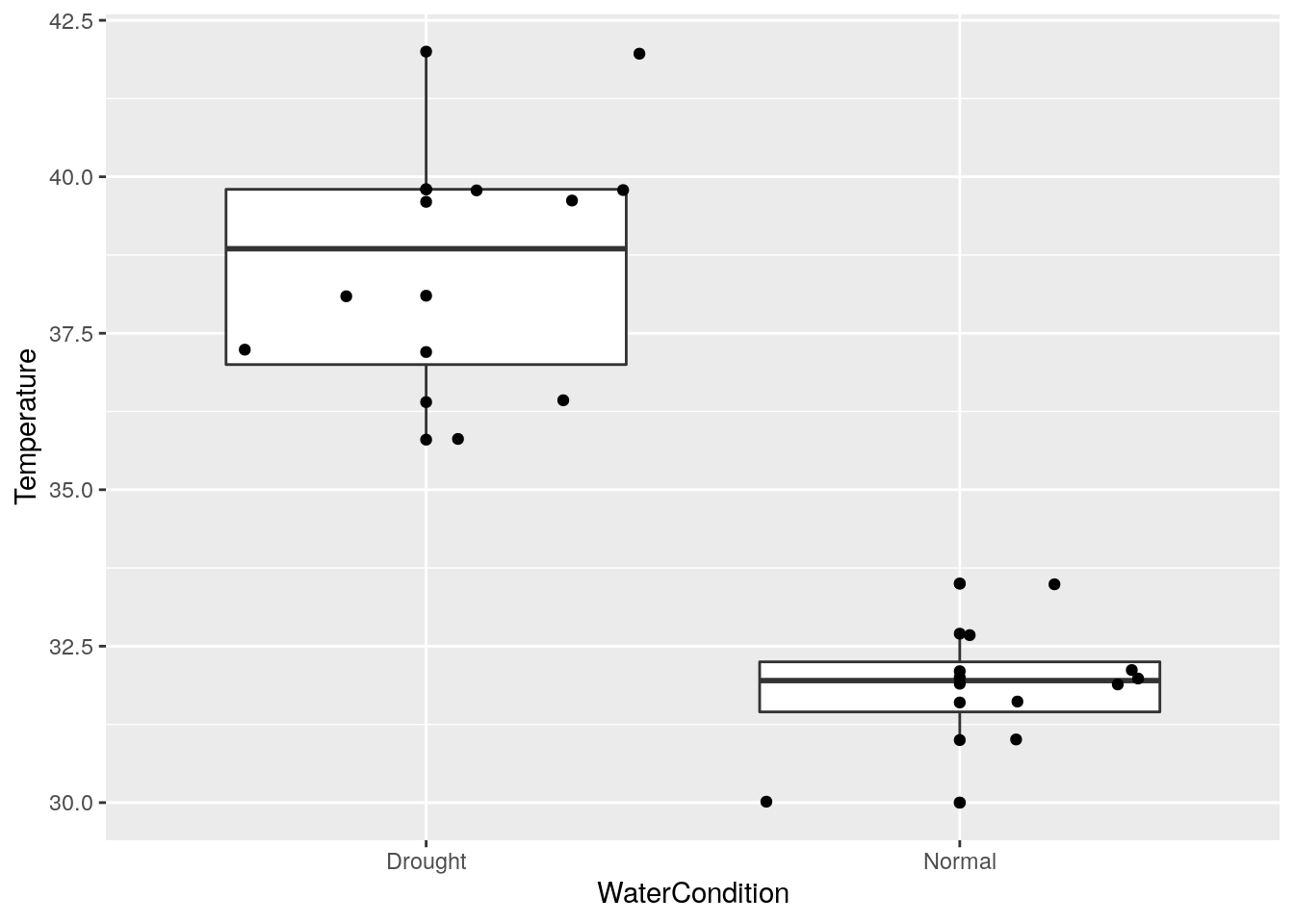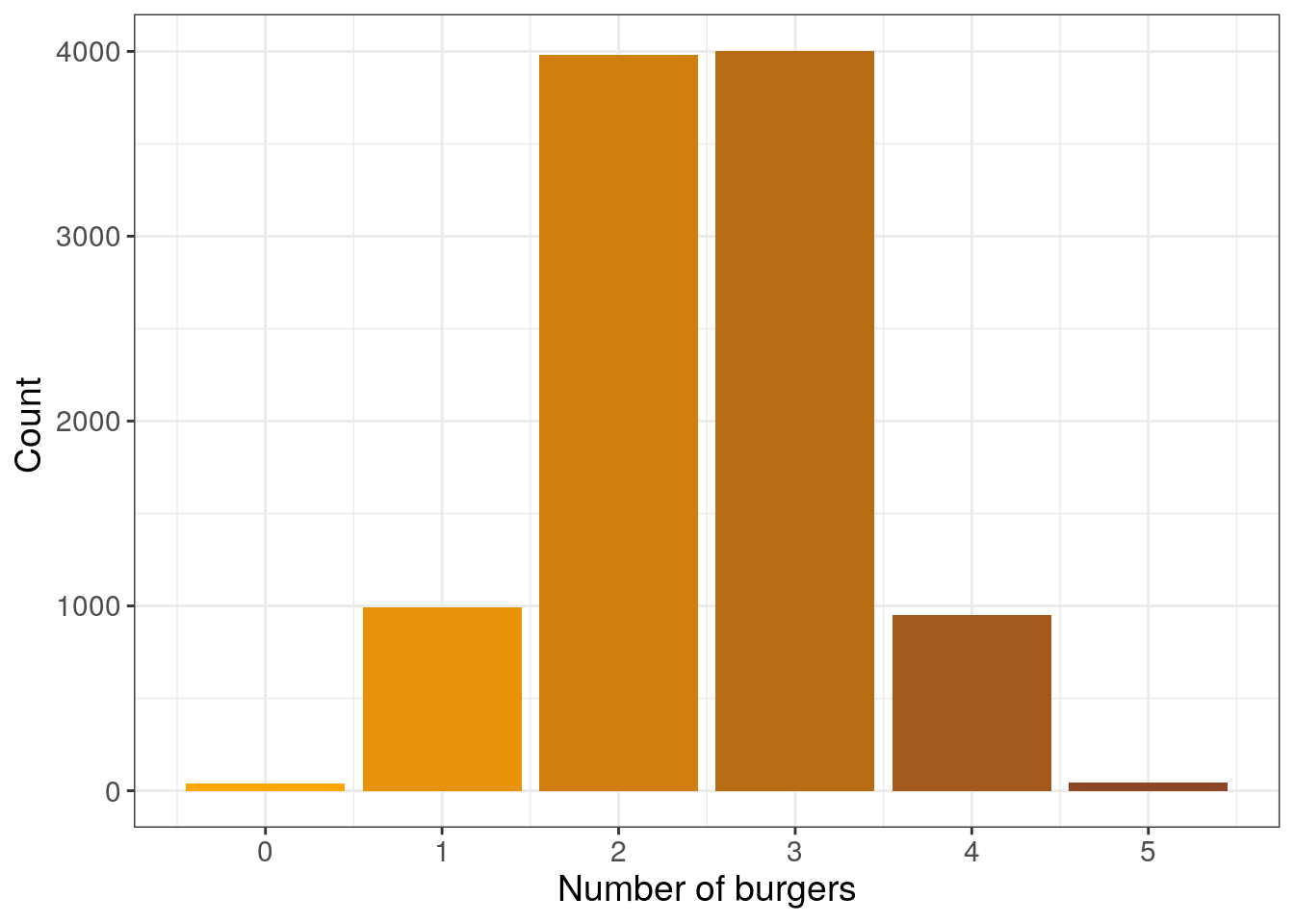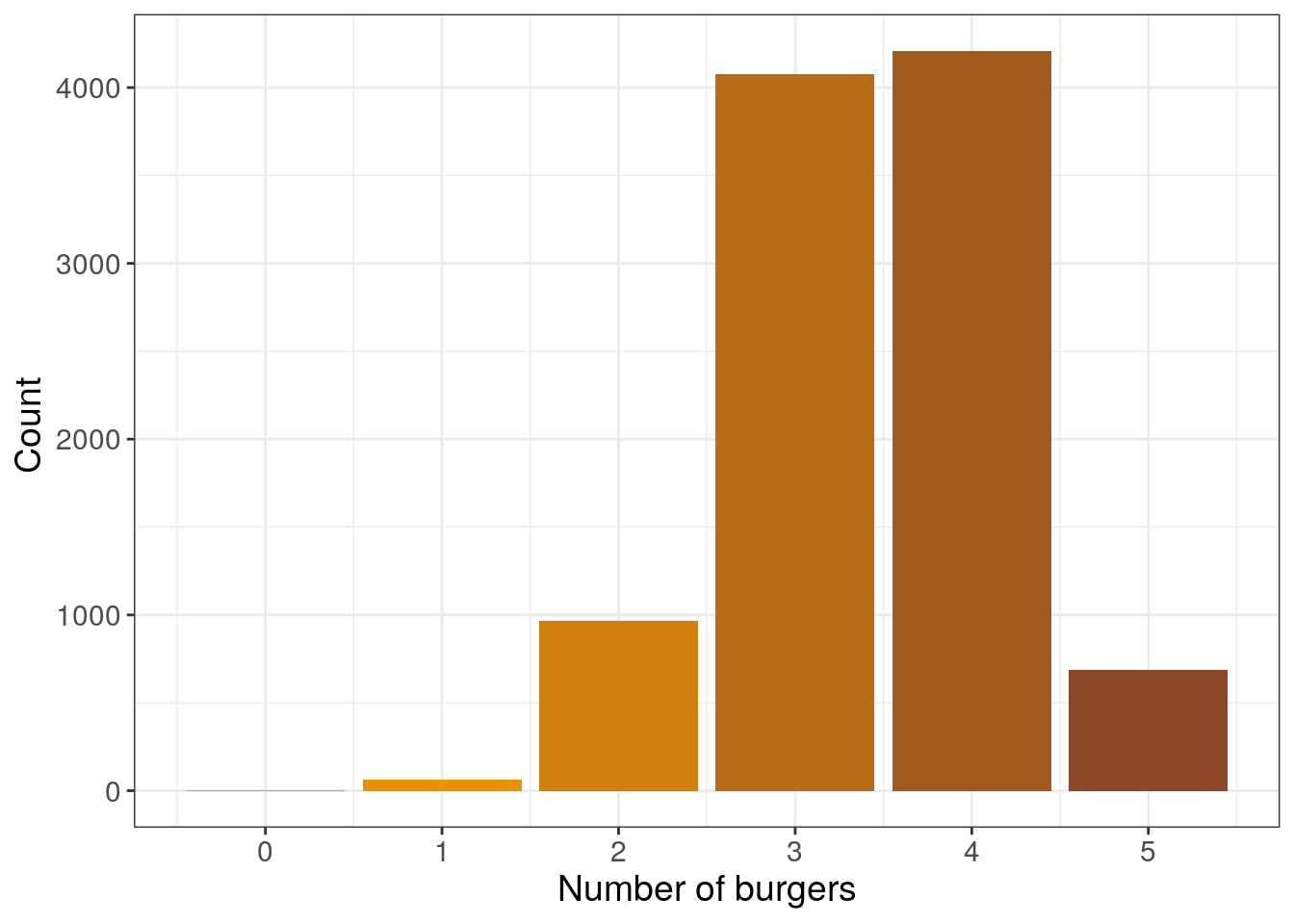Today:

• overview of key ideas about modelling.
• understand the function lm()
• know basic assumptions of linear models and how to assess them

## Visual example

Does leaf temperature vary based on water stress in a wild type tomato?

``````# simple comparison model
destfile = "data/droughtdata.csv")

str(drought_data)``````
``````## 'data.frame':    32 obs. of  4 variables:
##  \$ plant         : int  1 1 1 1 2 2 2 2 3 3 ...
##  \$ Genotype      : Factor w/ 2 levels "mutant","WT": 2 2 2 2 2 2 2 2 1 1 ...
##  \$ WaterCondition: Factor w/ 2 levels "Drought","Normal": 2 2 2 2 2 2 2 2 2 2 ...
##  \$ Temperature   : num  30 31 31.6 31.9 32 32.1 32.7 33.5 30 31 ...``````
``````wt_data <- drought_data[drought_data\$Genotype=="WT",]

library(ggplot2)
library(plyr)
ggplot(wt_data, aes(x=WaterCondition, y=Temperature)) +
geom_boxplot() + geom_point() + geom_jitter()````````````ddply(.data = wt_data, .variables = c("WaterCondition"),
.fun = function(x){summary(x\$Temperature)})``````
``````##   WaterCondition Min. 1st Qu. Median    Mean 3rd Qu. Max.
## 1        Drought 35.8   37.00  38.85 38.5875   39.80 42.0
## 2         Normal 30.0   31.45  31.95 31.8500   32.25 33.5``````

No overlap at all between the temperatures of water conditions. Could this happen “by chance”?

To answer that we need implicitly or explicitly a model of the biological variation that generated these data.

A T-test? Sure, that works in this case.

``````t.test(x = wt_data[wt_data\$WaterCondition == "Drought", "Temperature"],
y= wt_data[wt_data\$WaterCondition == "Normal", "Temperature"])``````
``````##
##  Welch Two Sample t-test
##
## data:  wt_data[wt_data\$WaterCondition == "Drought", "Temperature"] and wt_data[wt_data\$WaterCondition == "Normal", "Temperature"]
## t = 8.1716, df = 10.354, p-value = 7.85e-06
## alternative hypothesis: true difference in means is not equal to 0
## 95 percent confidence interval:
##  4.908888 8.566112
## sample estimates:
## mean of x mean of y
##   38.5875   31.8500``````

But what model is that? What does it assume?

A t-test is a linear model and can be written as:

response variable = baseline + difference*(treatment == 1) + noise ; noise Normally distributed, independent

## What is a statistical model

What is a model?

• Formal but simplified representation of the world / an experiment
• If the representation is good enough for our goal…
• … we can learn something about the world from data

For instance a map is a model. It can have more or less details, different type of information, and be useful for different goals. For instance, using a world map or oceanic currents you could make sense of data about genetic structure in some algea species, because… but maybe you won’t explain everything because algea travel also attached to boat hulls; so maybe you would need a map of major maritime roads too.

### Digression on good and bad models

Candies practical: https://timotheenivalis.github.io/data/candies.mp4

Naive distribution from a model that assumes items all have the same probability to be picked:

``````dat <- data.frame(burgers = rhyper(nn = 10000, m=5, n = 5, k = 5))

colfunc <- colorRampPalette(c("#FFA500", "#8B4726"))

ggplot(dat, aes(x=burgers, color=burgers)) +
geom_histogram(binwidth=0.5, stat="count",fill = colfunc(6)) +
xlab("Number of burgers")+
ylab("Count ") +
scale_x_continuous(breaks = 0:5)+ theme_bw()+  theme(text = element_text(size=14))``````
``## Warning: Ignoring unknown parameters: binwidth, bins, pad``Create a function that generates a distribution corresponding to a better model where a type of item is more likely to be picked.

``````fancyhyperg <- function(burger = 5, fruit = 5, meals = 5, relativeprob = 2)
{
for(i in 1:meals)
{
meal <- sample(x = c(rep("burger", times=burger), rep("fruit", times=fruit)),
size = 1, prob = c(rep(relativeprob, burger), rep(1, fruit)))
ifelse(meal=="burger", yes = burger <- burger - 1,
no = fruit <- fruit - 1)
}
nb_burger_meals <- meals - burger
return(nb_burger_meals)
}``````

Use this function to generate data where the burger items are 3 times more likely to be picked:

``````x <- c(0,1,replicate(n = 10000, expr = fancyhyperg(relativeprob = 3)))

dat <- data.frame(burgers = x)

colfunc <- colorRampPalette(c("#FFA500", "#8B4726"))

ggplot(dat, aes(x=burgers, color=burgers)) +
geom_histogram(binwidth=0.5, stat="count",fill = colfunc(6)) +
xlab("Number of burgers")+
ylab("Count ") +
scale_x_continuous(breaks = 0:5)+ theme_bw()+  theme(text = element_text(size=14))``````
``## Warning: Ignoring unknown parameters: binwidth, bins, pad``## “All stats are lm”, Fitting linear models in R

You may have learned statistics with this type of flow charts: